Question

# Consider the system shown in the single-line diagram of Figure 3. All reactances are shown in...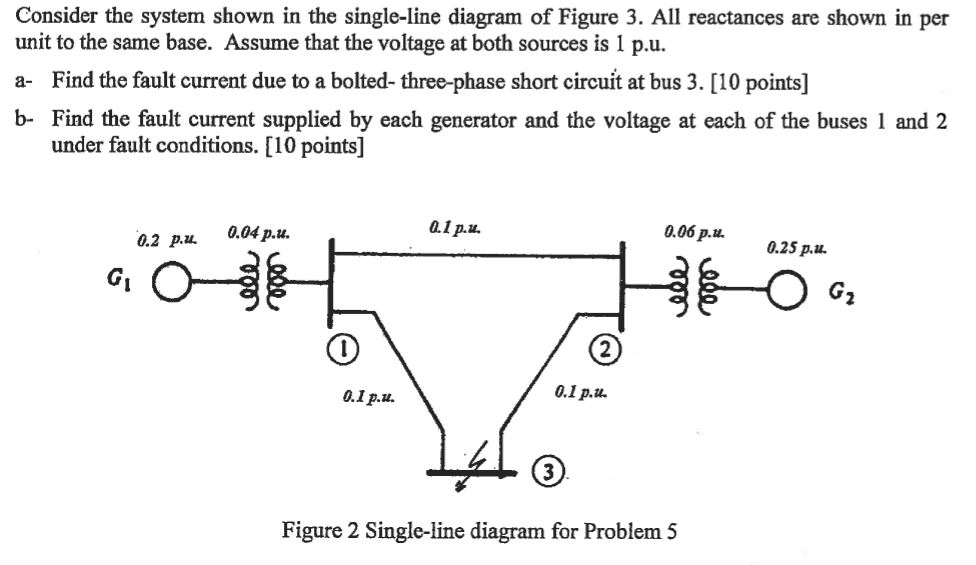Consider the system shown in the single-line diagram of Figure 3. All reactances are shown in per unit to the same base. Assume that the voltage at both sources is 1 p.u. a- Find the fault current due to a bolted- three-phase short circuit at bus 3. [10 points] b- Find the fault current supplied by each generator and the voltage at each of the buses 1 and 2 under fault conditions. [10 points] 0.1 p.u 0.06 ?.u. 0.25 p.11. 0.2 p.u 0.04p.u. 0.1 p.u 0.1 p.u. Figure 2 Single-line diagram for Problem 5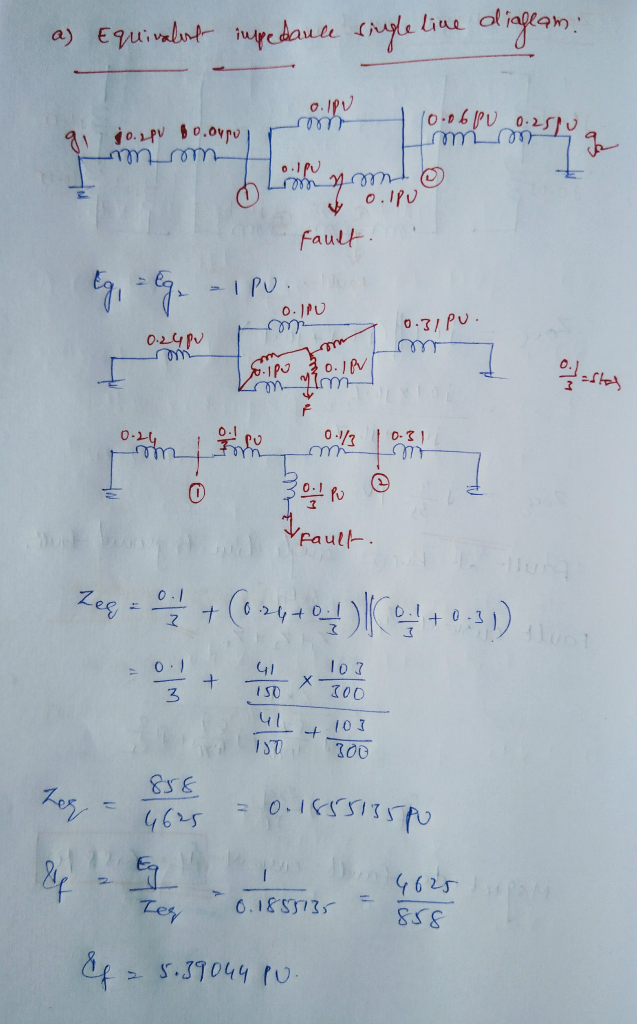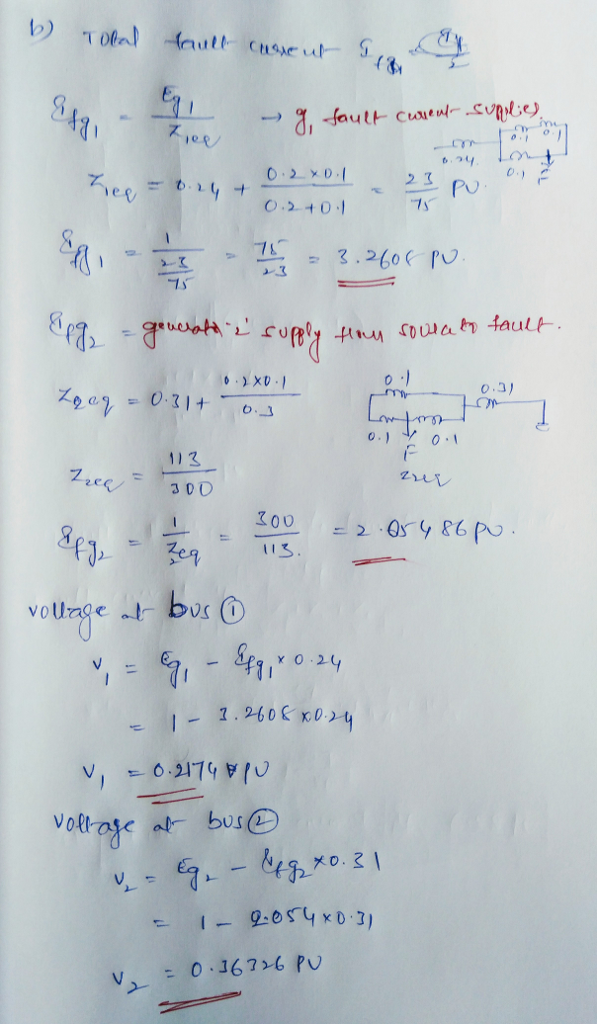#### Earn Coins

Coins can be redeemed for fabulous gifts.

Similar Homework Help Questions
• ### Consider the system shown in the single-line diagram of Figure 3. All reactances are shown in...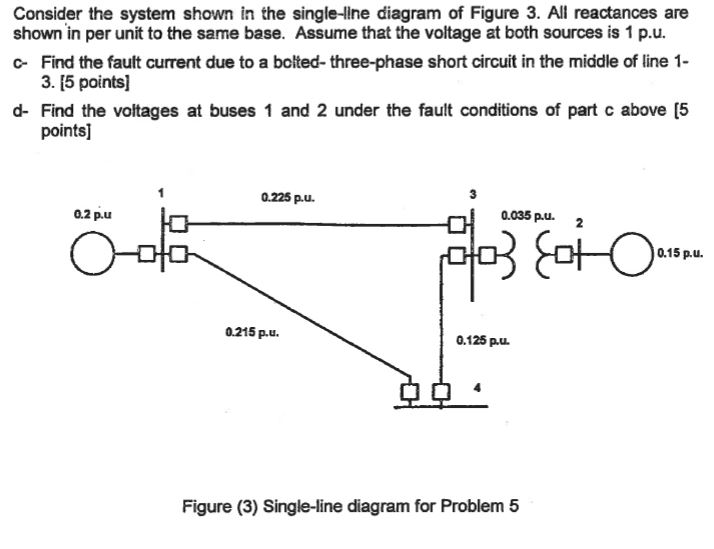Consider the system shown in the single-line diagram of Figure 3. All reactances are shown in per unit to the same base. Assume that the voltage at both sources is 1 p.u. c- Find the fault current due to a bolted-three-phase short circuit in the middle of line 1- 3. [5 points d- Find the voltages at buses 1 and 2 under the fault conditions of part c above [5 points] 0.225 pu. 0.035 pu. 0.2p.u ? 0.15 p.u. 0.215...

• ### Consider the system Shown in the single-line diagram of Figure (3). All reactance are shown in per unit to the same base. Assume that the voltage at both sources is 1 p.u.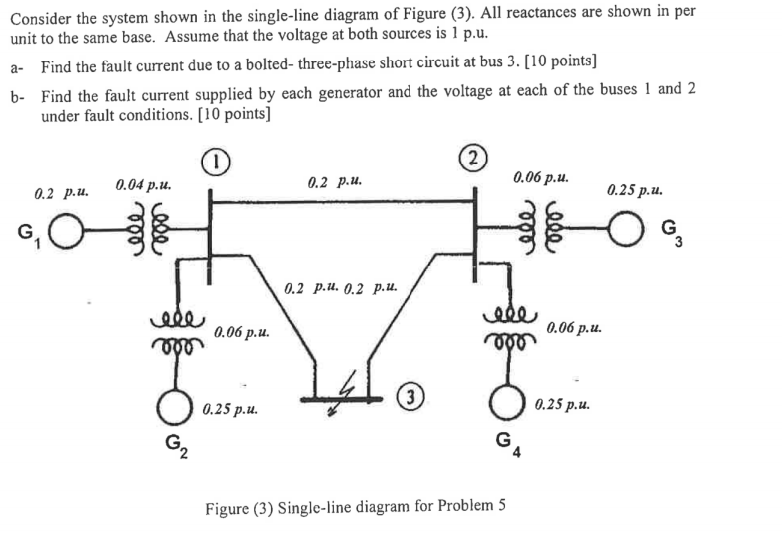Consider the system shown in the single-line diagram of Figure (3). All reactances are shown in per unit to the same base. Assume that the voltage at both sources is $$1 \mathrm{p}$$.u. a- Find the fault current due to a bolted- three-phase short circuit at bus $$3 .[10$$ points $$]$$ b- Find the fault current supplied by each generator and the voltage at each of the buses 1 and 2 under fault conditions. $$[10$$ points $$]$$

• ### PROBLEM 5 a Discuss the consequences of short circuit faults on electric power systems [5 Points...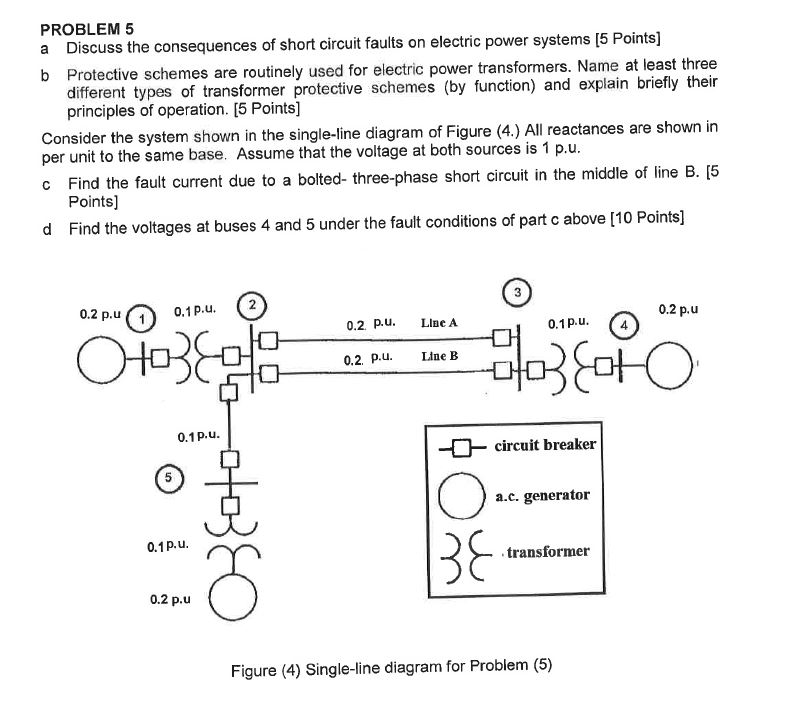PROBLEM 5 a Discuss the consequences of short circuit faults on electric power systems [5 Points Protective schemes are routinely used for electric power transformers. Name at least three different types of transformer protective schemes (by function) and explain briefly their principles of operation. [5 Points] Consider the system shown in the single-line diagram of Figure (4 per unit to the same base. Assume that the voltage at both sources is 1 p.u .) All reactances are shown in ind...

• ### 15 (c) Consider the single-line diagram of the power system shown in Figure. Neglect all resistances,...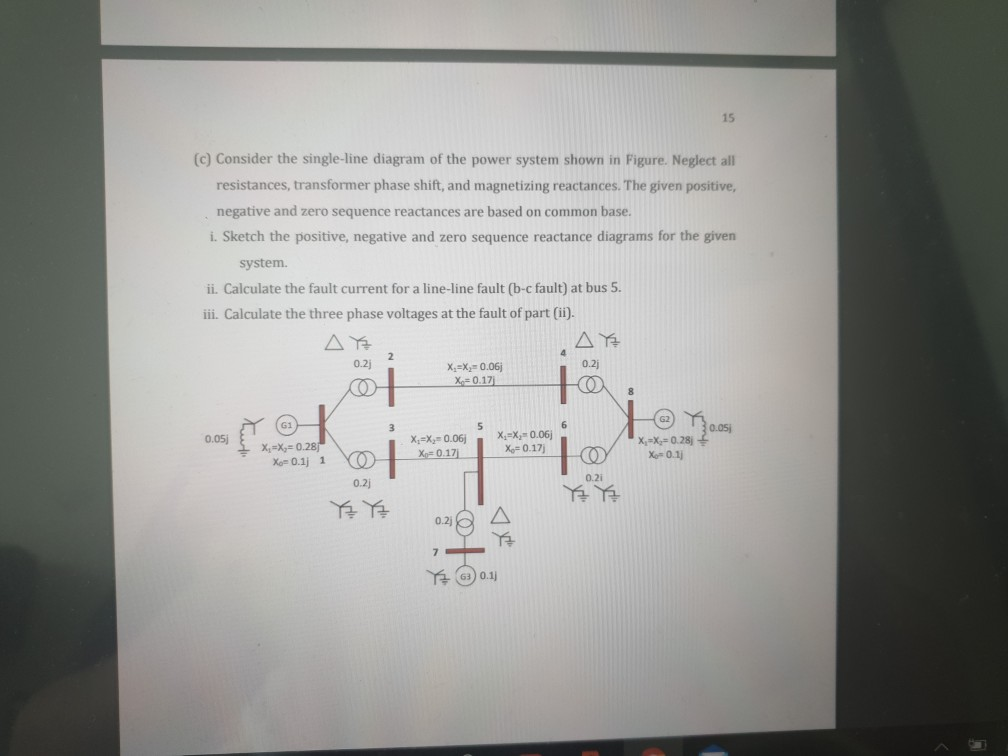15 (c) Consider the single-line diagram of the power system shown in Figure. Neglect all resistances, transformer phase shift, and magnetizing reactances. The given positive, negative and zero sequence reactances are based on common base. i. Sketch the positive, negative and zero sequence reactance diagrams for the given system. ii. Calculate the fault current for a line-line fault (b-c fault) at bus 5. iii. Calculate the three phase voltages at the fault of part (ii). AE AE 0.2) X.=X=0.06 X=0.17j...

• ### Figure 1 Single line diagram b2 b3 b1 b4 grid Τι 13 A power system single line diagram is shown...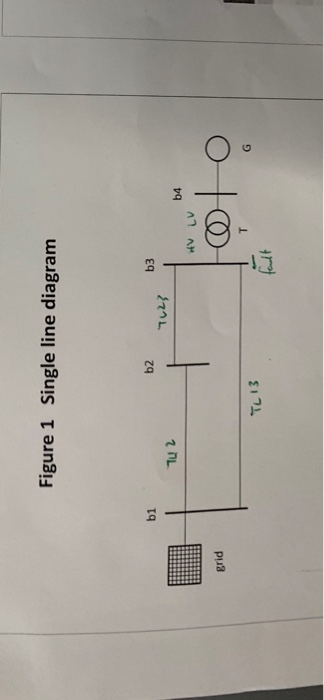Figure 1 Single line diagram b2 b3 b1 b4 grid Τι 13 A power system single line diagram is shown in Figure 1. The single line diagram shows a synchronous generator G connected to a large 50 Hz grid via its unit transformer T and a network of three transmission lines. Relevant details of the grid, transformer, generator and overhead lines are provided in Tables I,II,II & IV respectively. A double line to ground fault occurs at bus 3 Questions....

• ### The single-line diagram of a four-bus system and its bus impedance matrix are shown below BUS...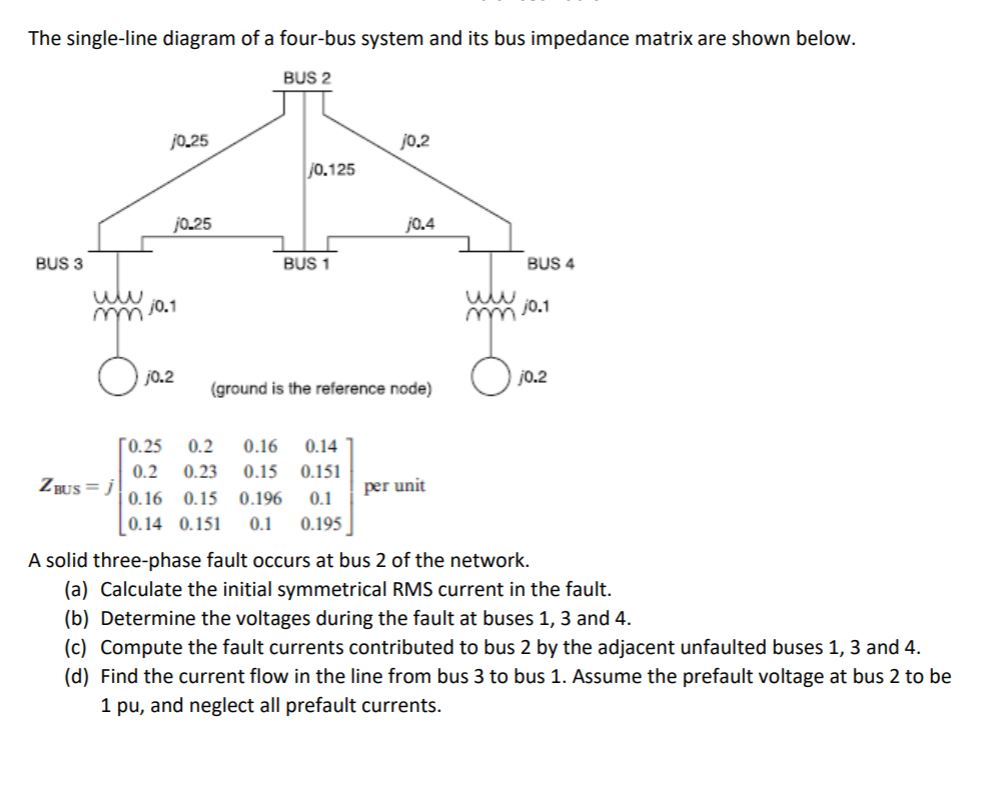The single-line diagram of a four-bus system and its bus impedance matrix are shown below BUS 2 0.25 j0.2 0.125 0.25 0.4 BUS 3 BUS 1 BUS 4 j0.1 0.1 j0.2 j0.2 ground is the reference node) 0.25 0.2 0.16 0.14 0.2 0.23 0.15 0.151 ZBUs =기0.16 0.15 0.196 0.1 0.14 0.151 0. 0.195 A solid three-phase fault occurs at bus 2 of the network. (a) Calculate the initial symmetrical RMS current in the fault. (b) Determine the voltages during...

• ### A single line diagram of a power system is shown in Fig. 2. The system data with equipment ratings and assumed sequence reactances are given the following table. The neutrals of the generator and A-Y...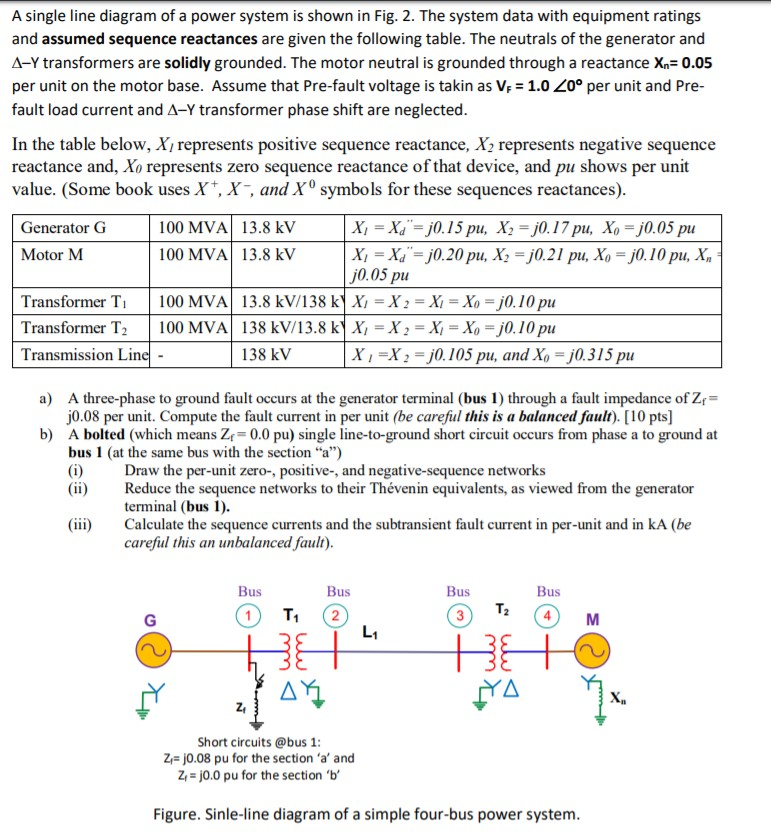A single line diagram of a power system is shown in Fig. 2. The system data with equipment ratings and assumed sequence reactances are given the following table. The neutrals of the generator and A-Y transformers are solidly grounded. The motor neutral is grounded through a reactance Xn 0.05 per unit on the motor base. Assume that Pre-fault voltage is takin as VF-1.0 ,0° per unit and Pre- fault load current and Δ-Y transformer phase shift are neglected In the...

• ### The single-line diagram of a three-phase power system is shown. Equipment ratings are given as follows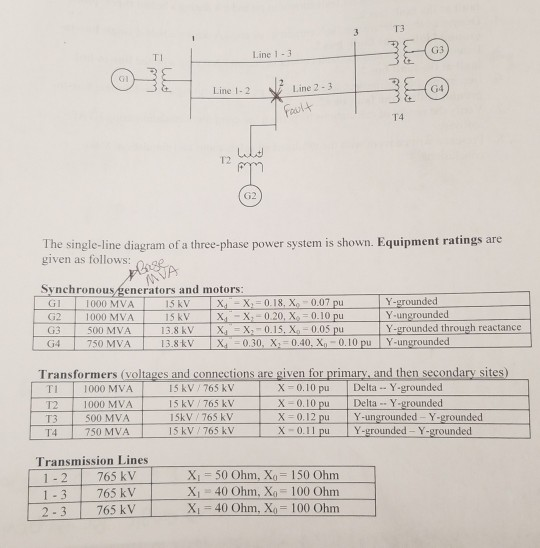The single-line diagram of a three-phase power system is shown. Equipment ratings are given as follows: The inductor connected to generator 3 neutral has a reactance of $$0.05$$ pu using generator 3 ratings as a base.1. Draw the zero-, positive-, and negative -sequence  reactance diagrams using a $$1000 \mathrm{MVA}, 765 \mathrm{kV}$$ base in the zone of line $$1-2$$.2. Faults at bus 2 are of interest. Determine the Thevenin equivalent of each sequence network as viewed from the fault bus. Prefault voltage...

• ### The one-line diagram of a simple power system is shown in Figure 1. The neutral of...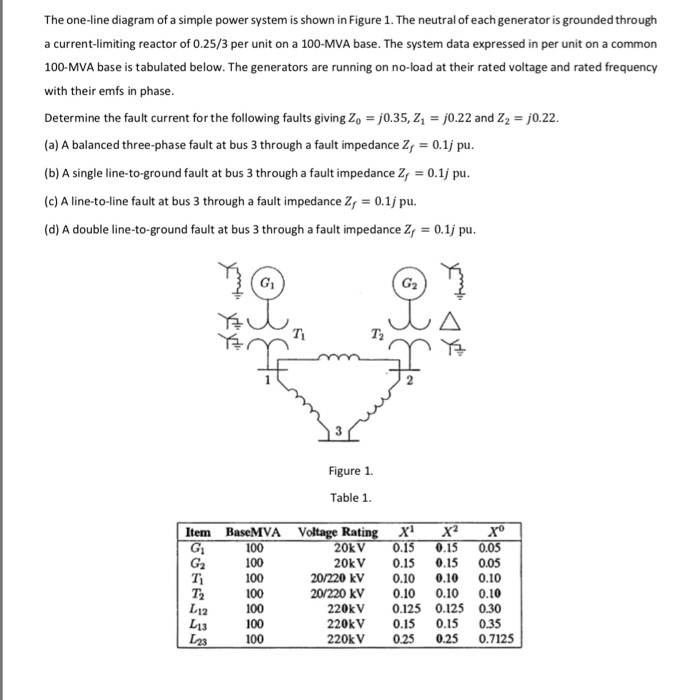The one-line diagram of a simple power system is shown in Figure 1. The neutral of each generator is grounded through a current-limiting reactor of 0.25/3 per unit on a 100-MVA base. The system data expressed in per unit on a common 100-MVA base is tabulated below. The generators are running on no-load at their rated voltage and rated frequency with their emfs in phase. Determine the fault current for the following faults giving Zo = 0.35, Z = 0.22...

• ### The following figure shows the one-line diagram of a single power network which has the line...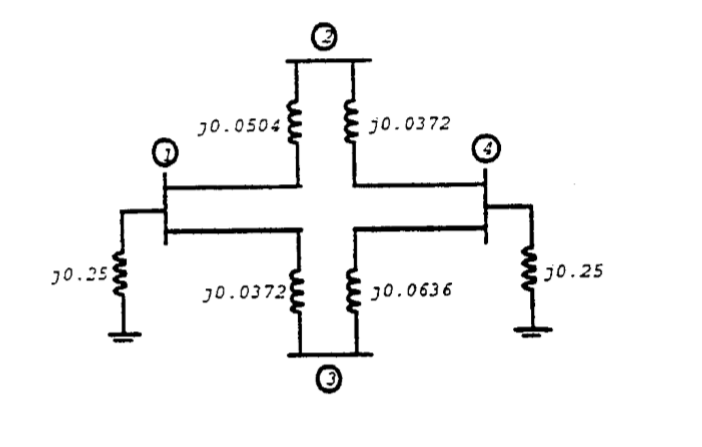The following figure shows the one-line diagram of a single power network which has the line admittances shown on the figure. Each generator connected to buses 1 and 4 has a sub-transient reactance of 0.25 pu. Determine for the network a. Ybus b. Zbus c. The sub-transient current in per unit in a three-phase fault on bus 3 and d. The contributions to the fault current from line 1-3 and from 4-3 ૩૦.૦૬૦-૬ ૬ . ૦૩72 , 30.23 90.0372૬ ૬૦.૦૦૩%...